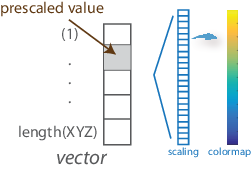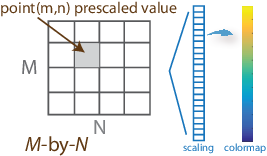# view

Display point cloud

## Syntax

``view(player,ptCloud)``
``view(player,xyzPoints)``
``view(player,xyzPoints,color)``
``view(player,xyzPoints,colorMap)``

## Description

example

````view(player,ptCloud)` displays a point cloud in the `pcplayer` figure window, `player`. The points, locations, and colors are stored in the `ptCloud` object.```
````view(player,xyzPoints)` displays the points of a point cloud at the locations specified by the `xyzPoints` matrix. The color of each point is determined by the z value.```
````view(player,xyzPoints,color)` displays a point cloud with colors specified by `color`.```
````view(player,xyzPoints,colorMap)` displays a point cloud with colors specified by `colorMap`.```

## Examples

collapse all

`ptCloud = pcread('teapot.ply');`

Define a rotation matrix and 3-D transform.

```x = pi/180; R = [ cos(x) sin(x) 0 0 -sin(x) cos(x) 0 0 0 0 1 0 0 0 0 1]; tform = affine3d(R);```

Compute x-_y_ limits that ensure that the rotated teapot is not clipped.

```lower = min([ptCloud.XLimits ptCloud.YLimits]); upper = max([ptCloud.XLimits ptCloud.YLimits]); xlimits = [lower upper]; ylimits = [lower upper]; zlimits = ptCloud.ZLimits;```

Create the player and customize player axis labels.

```player = pcplayer(xlimits,ylimits,zlimits); xlabel(player.Axes,'X (m)'); ylabel(player.Axes,'Y (m)'); zlabel(player.Axes,'Z (m)');```Rotate the teapot around the z-axis.

```for i = 1:360 ptCloud = pctransform(ptCloud,tform); view(player,ptCloud); end```## Input Arguments

collapse all

Point cloud, specified as a `pointCloud` object. The object contains the locations, intensities, and RGB colors to render the point cloud.

Point Cloud PropertyColor Rendering Result
`Location` only Maps the z-value to a color value in the current color map.
`Location` and `Intensity`Maps the intensity to a color value in the current color map.
`Location` and `Color`Use provided color.
`Location`, `Intensity`, and `Color`Use provided color.

Player for visualizing 3-D point cloud data streams, specified as a `pcplayer` object.

Point cloud x, y, and z locations, specified as either an M-by-3 or an M-by-N-by-3 numeric matrix. The M-by-N-by-3 numeric matrix is commonly referred to as an organized point cloud. The `xyzPoints` numeric matrix contains M or M-by-N [x,y,z] points. The z values in the numeric matrix, which generally correspond to depth or elevation, determine the color of each point.

Point cloud color of points, specified as one of:

You can specify the same color for all points or a different color for each point. When you set `color` to `single` or `double`, the RGB values range between [0, 1]. When you set `color` to `uint8`, the values range between [0, 255].

Points InputColor SelectionValid Values of `C`
`xyzPoints`Same color for all points

1-by-3 RGB vector, or the short or long name of a MATLAB `ColorSpec` colorDifferent color for each pointM-by-3 matrix or M-by-N-by-3 matrix containing RGB values for each point.Point cloud color of points, specified as one of:

• M-by-1 vector

• M-by-N matrix

Points InputColor SelectionValid Values of `C`
`xyzPoints`Different color for each pointVector or M-by-N matrix. The matrix must contain values that are linearly mapped to a color in the current `colormap`.